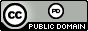시간 제한메모리 제한제출정답맞힌 사람정답 비율
2 초 512 MB123382827.723%

## 문제

You may have heard of the “maximum subarray problem” from your university’s undergraduate algorithms course. The problem goes like this: You are given an array A of n integers, and the task is to find a contiguous subarray of A whose sum is maximum. For example, in the array below:

A := [-2, 3, 5, -7, 8, 13, -20, 14, 1],

the solution is the subarray from the second index (the number 3) to the sixth index (the number 13), with total sum 22. The problem can be solved via divide and conquer, in O(n log n) time, or dynamic programming, in O(n) time.

Being a strong student in algorithms yourself, you are of course familiar with both approaches, so we won’t bother explaining them here. However, your classmate Steve, a not-so-strong student in algorithms, has been bragging to you about the maximum subarray problem. He claims that not only can he solve the maximum subarray problem in linear time, he can even solve what he calls the “k-maximum subarray problem,” ALSO in linear time! What is the “k-Maximum subarray problem,” you ask? In a condescending tone, Steve explains: It is the natural generalization of the maximum subarray problem. Given an array A of n integers, you must find k disjoint, contiguous subarrays of A so that their total sum is maximum.

You’re not at all confident that Steve knows how to solve the “k-maximum subarray problem” in linear time, let alone that there even exists a linear time solution. You have gone to every algorithms lecture, however, a near-infinite improvement on Steve’s attendance, and so you believe if anyone is to be the authority on the problem, it probably should be you.

You decide to write a program to solve the “k-maximum subarray problem.” You can use this to verify Steve’s likely-incorrect algorithm. To simplify things, you make the program just output the value of an optimal solution. You can modify it later if you want the solution itself. Furthermore, you decide your algorithm is sufficiently efficient as long as its running time is bounded by a small polynomial in n and k. You think coming up with an actually-correct linear time solution would probably take you awhile, and you’ve decided you only want to spend, say, a maximum of five hours on your code.

## 입력

The input will consist of two lines. On the first line are the integers n and k, (1 ≤ k ≤ n ≤ 5 000). On the second line are n integers, representing the array A. The integers in array A will be between −109 and 109, inclusive.

## 출력

Output the maximum possible total sum of k disjoint contiguous subarrays of array A. Although the subarrays are required to be disjoint, a subarray may end at index i and another subarray start at index i + 1. No subarray is allowed to be empty.

## 예제 입력 1

9 1
-2 3 5 -7 8 13 -20 14 1


## 예제 출력 1

22


## 예제 입력 2

11 3
23 -10 12 -2 -33 13 -5 55 23 -8 13


## 예제 출력 2

126


## 출처• 문제를 만든 사람: Bryce Sandlund